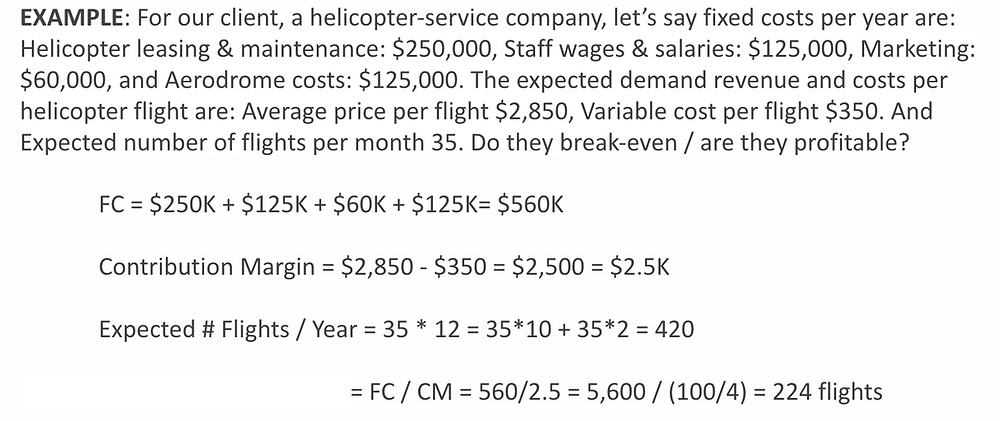Search
•Bryan Wang

# Useful Formulas, Equations, & Data-Points For Case Interviews

Market sizing is a common quantitative question in Market Entry cases. For Profitability cases, you instead are asked about the ‘Break-Even’ questions.

• Profit = Revenue (Price x Volume) – Costs (Fixed + Variable) Breakeven is the point at which Costs = Revenue. It is usually quantified in number of units. To find the breakeven point, you can use 2 equations: Breakeven units = Fixed Cost / Contribution Margin Revenue (re: Price x Volume) – Costs (re: Fixed Cost + Variable Cost) = 0

The first break-even formula is more commonly used. Set breakeven units as ‘x’ (where your goal is to find ‘x’). Contribution Margin is equal to Revenue per product sold minus Variable Cost per product sold. By dividing Fixed Costs by Contribution Margin, you will be able to find the number of units the client needs to sell in order for Revenues to equal Costs, therefore breaking-even.

AGAIN, reaching the break-even number is not what’s important — contextualizing, impacting, and communicating the “so what” of this number is what’s important. If the break-even number is 50 million (re: client needs to sell 50M units to reach zero in profits) and the client is a small-time manufacturer, then there is a clear problem. It can’t hit its break-even goals, which is a strong sign they should exit the business / product segment. If the break-even number is more reasonable, however, then this is encouraging — it means the client can achieve profitability with this product segment, and the numbers paint a sign that they should pursue launching said product.

Here’s an example of what a break-even question might look like:Since the break-even point is 224 flights, it’s clear that our client is a profitable business (re: 420 expected flights > 224 break-even point ). This is a clear sign they should continue the business, as it is a robust one.

Another key equation, ‘Elasticity’, is used to solve ‘Pricing’ cases. When determining a price that will optimize revenues and therefore profits, it’s important to note that, in some cases, decreasing the price will actually lead to optimal revenues. Identifying the elasticity of your client’s products is key, therefore, to solving their pricing questions.### Some Quick Data Points to Note

U.S. Population -> 300M, 320M, 330M, anywhere in this ball park (I personally use 320M, as it’s easier to play around with 25%’s)

China Population -> 1B, 1.2B (for the same reason above)

UK Population -> 60M

World Population -> 6B, 7B

Average Household Number -> 3 individuals per household

Currency Conversions -> 1 USD = 7 RMB, 1 USD = 0.75 Pounds, 1 USD = 0.85 Euro’s

Volume of a sphere = 4/3 π x the cube of its radius

Volume of a cube = length x width x height

Volume of a pyramid = 1/3 x base squared x height

5280 feet in a mile, 1000 meters in a kilometer

A short city block is 1/8 of a mile, a long block is 1⁄4 mile

A football field is 100 yards long